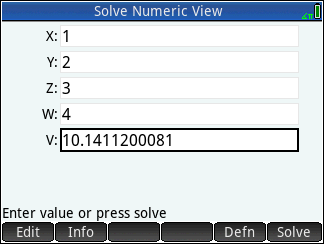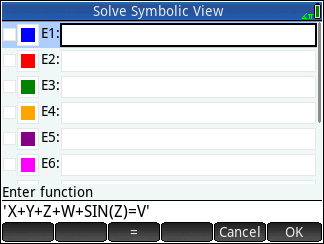cancel
Showing results for
Did you mean:

### Note on archived topics.

This topic has been archived. Information and links in this thread may no longer be available or relevant.
If you have a question create a new topic by clicking here and select the appropriate board.
Level 1
7 4 0 0
Message 1 of 11
11,473
Flag PostHP Recommended

# How does Hp prime graphing calculator solve equations, X Y Z W=V ?

I have purchased HP Prime Graphing, 35s and 28s calculators for my projects.  I cannot figure out how the Prime Graphing one does equations such as X+Y+Z+W+Sin(Z)=V.

Very best regards,

Benjamin

Tags (2)
10 REPLIES 10
Level 8
527 514 90 161
Message 2 of 11
11,467
Flag PostHP Recommended

## How does Hp prime graphing calculator solve equations, X Y Z W=V ?

There are several solvers in the HP Prime, but the kind you're looking for is probably the built in "Solver App".  Just press Apps, tap the Solve app icon.  In the Solve Symbolic View, type your equation into one of the ten slots, like this:Then press Num.  Enter the known values, highlight the unknown value, and tap Solve.  Here's your equation solved for V with some sample inputs:Of course, be sure to set any modes that your equation depends on, e.g. Degree or Radian mode.  This can be done globally in Home Settings, or locally for each app in Symb Setup.

Hope that helps!

-Joe-
Level 1
7 4 0 0
Message 3 of 11
11,456
Flag PostHP Recommended

## How does Hp prime graphing calculator solve equations, X Y Z W=V ?

Hello dear Joe,
Thank you for your respond. I have tried all the possible ways that I could think of, but it seems that there is a problem with the calculator software, or it is defective. I tried the very same procedure that you have indicated. As soon as I input for example X+Y "Enter", it gives me Error: Bad argument count!
Also, in Function Symbolic View mode, if I Enter X+2, it gives me Error: Bad argument count.
Very best regards,
Benjamin
Level 9
970 953 119 185
Message 4 of 11
11,450
Flag PostHP Recommended

## How does Hp prime graphing calculator solve equations, X Y Z W=V ?

Hi,

If you are in RPN mode, use the  ' ' [shifted ( ) key] when entering equations, because in RPN mode equations are algebraic expressions that require to be in ' ' marks.

HTH

-Bart
_________________________________________________________
calculator enthusiast
Level 8
527 514 90 161
Message 5 of 11
11,440
Flag PostHP Recommended

## How does Hp prime graphing calculator solve equations, X Y Z W=V ?

Sorry, I assumed that you were in either Textbook Entry mode (the default), or Algebraic Entry mode.  As Bart said, you'll have to surround your equation with single-quote marks if tyou're in RPN Entry mode, as in the edit line of this screen capture:(Good catch, Bart!)

-Joe-
Level 1
7 4 0 0
Message 6 of 11
11,424
Flag PostHP Recommended

## How does Hp prime graphing calculator solve equations, X Y Z W=V ?

Hello dear Joe,

I may need to explain more what I need to do with this calculator.  I would like to find out if I could solve the equation/s by the information which has/have been provided by other equation/s, such as:
XxY-Z=V
2X+3Y-2Z=-2V
12X+Y-8Z=10V
16X+3Y+9Z=-49V, where V=-1
Then in a more complicated and larger scales.

P.S. Please note that people with HP Prime Graphic Calculators version V0.025.5106., need to contact the HP Technical Support Deartment, 800-HPINVENTS, and ask for the software/frameware up-date, there are some issues with these Versin-Calculators.

Very best regards, and have a great night,

Benjamin

Level 8
527 514 90 161
Message 7 of 11
11,422
Flag PostHP Recommended

## How does Hp prime graphing calculator solve equations, X Y Z W=V ?

Just put all your equations into separate slots in the Solver app, up to ten of them at a time.  You can save a copy of the Solver app so that you don't have to retype often-used sets of equations.  See Chapter 13 in the HP Prime User Guide ("Solve app"), especially the section entitled "Several equations" (starts on page 263 in my copy; your version may differ).

Just remember that the solver (accessed by pressing Num) works with whatever equations have a checkmark in the Symbolic View (accessed by pressing Symb) and ignores the others.  Sometimes you will want to start with only one equation checked, solve for a variable, then add more equations and solve for more variables, and so on, until they are all solved for.  If you have a coherent system of equations, however, you can check them all right from the start.

-Joe-
Level 1
15 11 0 0
Message 8 of 11
4,813
Flag PostHP Recommended

## How does Hp prime graphing calculator solve equations, X Y Z W=V ?

Great explanation. Simple question I hope you or some one can solve; Given the same example you just explained here, how can I use it in a custom program?

At the front end, you'd INPUT known values for variables, then run it through the solver, then TEXTOUT_P the number that equals 'X'.

Level 6
305 297 32 75
Message 9 of 11
4,779
Flag PostHP Recommended

## How does Hp prime graphing calculator solve equations, X Y Z W=V ?

A possible program would be:

```EXPORT MYPROGRAM()
BEGIN

INPUT({X,Y,Z,W,V});

A:=FNROOT(X+Y+Z+W+SIN(Z)=V,X,X);

RECT_P();
TEXTOUT_P(A,100,100);
FREEZE;

END;```

Instead of FNROOT you could also use:

Solve.SOLVE, or, when the Solve app is active you can simply use: SOLVE.

The Solve app is active when you see at the top of the screen: Solve.

FNROOT is a general Home command and accessable via:

Toolbox (key B)-Math-Arithmatic-Find Root.

SOLVE is an app command and accessable via:

Toolbox-App-Solve-SOLVE.

Level 1
15 11 0 0
Message 10 of 11
4,737
Flag PostHP Recommended

## How does Hp prime graphing calculator solve equations, X Y Z W=V ?

Worked perfectly, Thank you!

### Note on archived topics.

This topic has been archived. Information and links in this thread may no longer be available or relevant.
If you have a question create a new topic by clicking here and select the appropriate board.
† The opinions expressed above are the personal opinions of the authors, not of HP. By using this site, you accept the Terms of Use and Rules of Participation